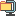검색어 입력폼

# [디지털 통신]홍익대 이호경 교수 디지털 통신 숙제 솔루션&족보

저작시기 2006.01 | 등록일 2006.02.21압축파일 (zip) | 9페이지 | 가격 1,000원

## 소개글

홍익대 이호경 교수 디지털 통신 숙제 1~5까지 솔루션 입니다.
그리고 중간 기말 시험 문제도 있습니다.

디지털 통신 숙제 풀이
기말고사
중간고사

## 본문내용

디지털 통신 HW #1 (Preliminary) 제출일 : 9월 30일 (월)

Problem 1) The joint pdf of the random variables X, Y is given as
.
(a) Find and .
(b) Are X, Y statistically independent?
(c) Find P(X<1, Y<1).
(d) Determine the pdf of Y given that X=1 .
Problem 2) Let X(t) be a real valued stationary random process with auto correlation function .
a) Determine and sketch the power spectral density of X(t).
b) Determine the autocorrelation function and the power spectral density of .
c) Sketch the real part of the auto correlation function determined in part (b).
(Hint : )
Problem 3) Determine the noise equivalent bandwidth for the two filters having transfer functions given below:
(a) .
(b) .
Problem 4) A random variable X is defined by
.
The random variable Y is related to X by Y = 2 + 3X .
a) Determine E{X}, E{X2}, and .
b) Determine .
c) Determine E{Y}, E{Y2}, and .
Problem 5 :
a) Find the Fourier Transform of f(t) given in Figure(a).
b) Find the Fourier Transform of g(t) given in Figure(b) (Hint : Use the Convolution).
c) Find the Fourier Transform of the periodic signal s(t) given in Figure(c).
d) Find the exponential Fourier series of s(t).

없음

## 압축파일 내 파일목록

디지탈통신_숙제풀이_4.hwp
디지털_통신homework3solution.pdf
디지털통신Homework3.hwp
디지털통신Homework4.hwp
디지털통신Homework5.hwp
디지털통신Homework_6.hwp
기말고사(2004).hwp
디통HW_2_solution.pdf
디통_HW_5풀이.pdf
중간고사(2004).hwp
Homework_1.hwp
Homework_2.hwp
HW_1_solution.pdf